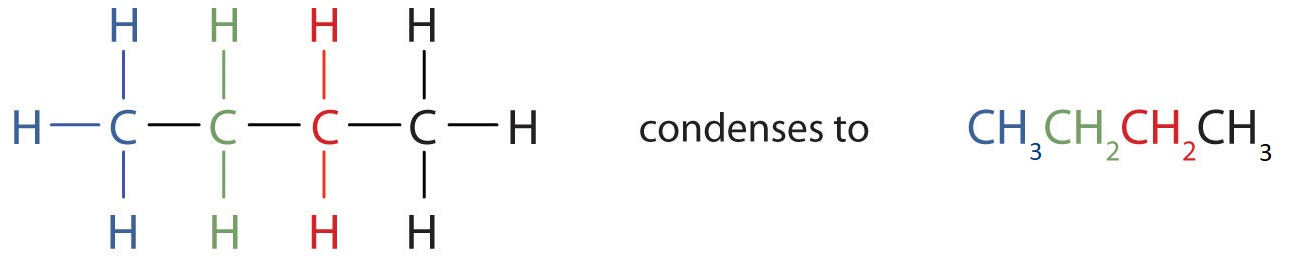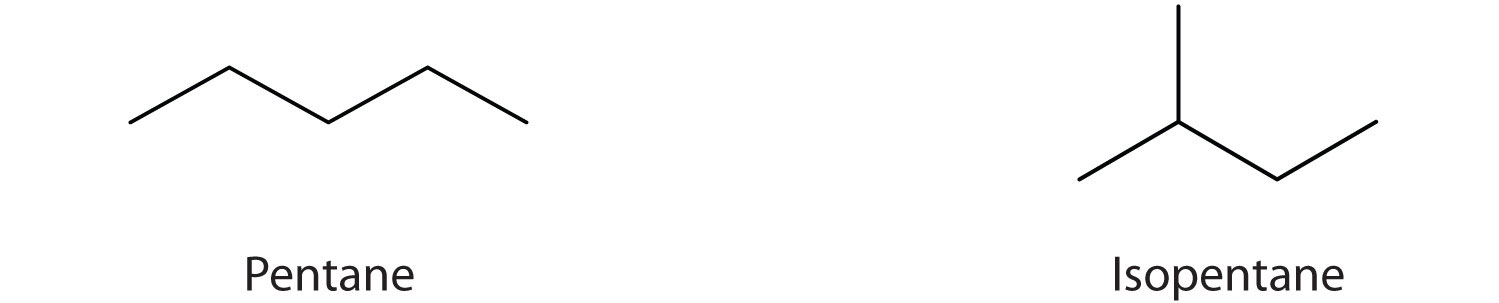# 12.4: Condensed Structural and Line-Angle Formulas

Skills to Develop

• Write condensed structural formulas for alkanes given complete structural formulas.
• Draw line-angle formulas given structural formulas.

We use several kinds of formulas to describe organic compounds. A molecular formula shows only the kinds and numbers of atoms in a molecule. For example, the molecular formula C4H10 tells us there are 4 carbon atoms and 10 hydrogen atoms in a molecule, but it doesn’t distinguish between butane and isobutane. A structural formula shows all the carbon and hydrogen atoms and the bonds attaching them. Thus, structural formulas identify the specific isomers by showing the order of attachment of the various atoms.

Unfortunately, structural formulas are difficult to type/write and take up a lot of space. Chemists often use condensed structural formulas to alleviate these problems. The condensed formulas show hydrogen atoms right next to the carbon atoms to which they are attached, as illustrated for butane:The ultimate condensed formula is a line-angle formula, in which carbon atoms are implied at the corners and ends of lines, and each carbon atom is understood to be attached to enough hydrogen atoms to give each carbon atom four bonds. For example, we can represent pentane (CH3CH2CH2CH2CH3) and isopentane [(CH3)2CHCH2CH3] as follows:Parentheses in condensed structural formulas indicate that the enclosed grouping of atoms is attached to the adjacent carbon atom.

## Key Takeaways

• Condensed chemical formulas show the hydrogen atoms (or other atoms or groups) right next to the carbon atoms to which they are attached.
• Line-angle formulas imply a carbon atom at the corners and ends of lines. Each carbon atom is understood to be attached to enough hydrogen atoms to give each carbon atom four bonds.